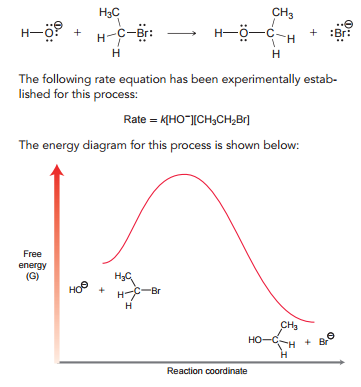# Problem: Consider the following reaction: (a) Draw the curved arrows showing a mechanism for this process.

93% (83 ratings)
###### Problem Details

Consider the following reaction:

(a) Draw the curved arrows showing a mechanism for this process.What scientific concept do you need to know in order to solve this problem?

Our tutors have indicated that to solve this problem you will need to apply the SN2 Reaction concept. You can view video lessons to learn SN2 Reaction. Or if you need more SN2 Reaction practice, you can also practice SN2 Reaction practice problems.

What is the difficulty of this problem?

Our tutors rated the difficulty ofConsider the following reaction: (a) Draw the curved arrows...as medium difficulty.

How long does this problem take to solve?

Our expert Organic tutor, Chris took 1 minute and 27 seconds to solve this problem. You can follow their steps in the video explanation above.

What professor is this problem relevant for?

Based on our data, we think this problem is relevant for Professor Daugulis' class at UH.

What textbook is this problem found in?

Our data indicates that this problem or a close variation was asked in Organic Chemistry - Klein 1st Edition. You can also practice Organic Chemistry - Klein 1st Edition practice problems.